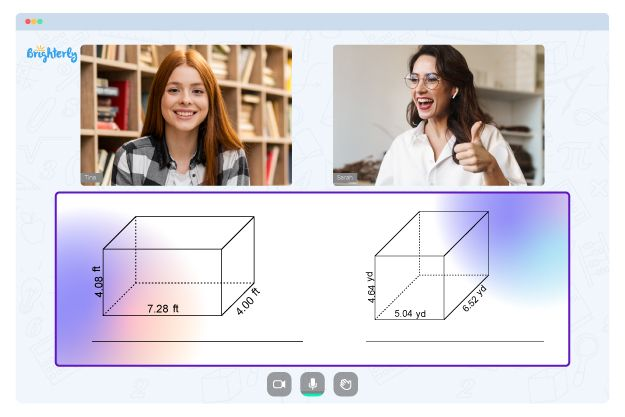# Area Of A Trapezoid Worksheets

are called bases, and the perpendicular distance between parallel sides is the height of the trapezoid. The form itself is well known in architecture as the main supporting structure for many forms of bridges.

These concepts can confuse 3rd graders, which is where an area of trapezoids worksheet comes in. The diagrams in the sheet help students determine the area of ​​different trapezoids using side lengths.

## What Is an Area of Trapezoid Worksheet?

An area of trapezoid worksheet usually contains simple geometric math exercises that will help students understand how to calculate the size of ​​a trapezoid. It explains how the formula for the area of ​​a trapezoid is similar to that of the triangle. Thought-provoking questions will encourage students to practice spatial reasoning and problem-solving skills.

Math for Kids

Is Your Child Struggling With Math?
1:1 Online Math Tutoring## Benefits of Using Area of Trapezoids Worksheet

A trapezoid area worksheet 3rd grade helps students develop their skills in finding the area of ​​a trapezoid using different methods. Working on the sheets, kids improve their analysis and problem-solving skills. Students gain a better idea of ​​how to deal with problems on trapezoids.### Area Of A Trapezoid Worksheets PDF

Area Of Trapezoid Worksheet### Area Of A Trapezoid Worksheets PDF

Area Of A Trapezoid Worksheet### Area Of A Trapezoid Worksheets PDF

Trapezoid Area Worksheet### Area Of A Trapezoid Worksheets PDF

Area Of Trapezoids Worksheet

The problems can be related to the direct application of the formula, the distance between parallel edges, the lack of a root, and other open issues. Children can download and print area of trapezoid worksheets, and exercises are free and easily used offline. Students can use the area of trapezoid worksheet answers to correct their own mistakes if necessary.

### Area and Perimeter Worksheets

Problems with Geometry?• Does your child struggle with understanding the concept of geometry?
• Try studying with an online tutor.

Is your child having trouble mastering geometry? An online tutor could provide the necessary guidance.